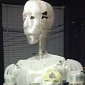# Joystick loop

Hi,

I'm having trouble to get my PS3 controller working as I want to.

My idea is to move the head when using the left stick.

Joypad is connected, it is detected and everything seems to work.

I want to move the head as long the stick is oblique.

This is the code (simplified withou servo, just prints):

joystick = Runtime.start("joystick","Joystick")
print(joystick.getControllers())
python.subscribe("joystick","publishJoystickInput")
joystick.setController(2)

def onJoystickInput(data):

# LEFT STICK
if (data.id == "x"):
if(data.value <= -0.5):
print ("Left stick: left (" + str(data.value) + ")")
elif(data.value >= 0.5):
print ("Left stick: right (" + str(data.value) + ")")
elif (data.id == "y"):
if(data.value <= -0.5):
print ("Left stick: up (" + str(data.value) + ")")
elif(data.value >= 0.5):
print ("Left stick: down (" + str(data.value) + ")")

The problem is that when the value of the joystick doesn't change anymore (eg: the stick is all inclined to the max inclination) the "onJoystickInput" is not called anymore. How can I still loop even if the values are not changed?

Thanks### This is the video, just in

This is the video, just in case https://photos.app.goo.gl/VoD3oHRUvGXOWSgs1### I think I found where the

I think I found where the problem is:

https://github.com/MyRobotLab/myrobotlab/blob/05ef7ca86b67623593e2e94089...

If the difference between old and new value is greater than a delta (0.0001), then the old value is updated.

I think a correct way to handle this would be at least set this delta to a variable, and have the possibility to set change it.

Something like this:

public float delta = 0;

public void setDelta(float delta) {

this.delta = delta;

}

public float getDelta() {

return this.delta;

}

// and use the variable accordingly:

if (Math.abs(input - component.value) > delta) { ...

I don't have eclipse/netbeans installed and so on, but the patch should be easy to add.

My 2 cents :)### velocity vs position

It sounds like you want to use the joystick to turn the inmoov head.  This is very do able without any code changes.

The reason the joystick doesn't publish all data points all the time even if they don't change is because it would simply be too much data coming back and forth.  So, the approach is only to publish the delta.. (i.e. what's changed.)

So, there are a few approaches to controlling servo positions with a joystick.

1. the joystick position is mapped from -1 to 1 to a range of 0 to 180 and this position is sent to the servo.  This means you need to hold the joystick in position for the servos to stay in that position.

2. the joystick position is mapped to a direction and speed of the servo while it's sweeping.  The more you push the joystick the faster it turns. when you let go of the joystick the servo stays in the position you left it in.

Is there some other approach you were thinking of ?

(There is a yet 3rd approach to servo position control.. and that is to connect the joystick to the input of the inverse kinematics 3d service, and allow the inverse kinematics to compute the angles for the servo.  In this case, the joystick is used to control a point in space and the inverse kinematics service would attempt to compute a solution that places the robots hand at that point in space... This is really an approach for controlling a robot arm with at least 3 servos connected to it.)

Here's some links to joystick / servo scripts that I've done.. their syntax is probably pretty far out of date.. but they might serve as some useful examples.

https://github.com/MyRobotLab/pyrobotlab/blob/master/home/kwatters/UberJ...

https://github.com/MyRobotLab/pyrobotlab/blob/master/home/kwatters/Joyst...

https://github.com/MyRobotLab/pyrobotlab/blob/master/home/kwatters/InMoo...### Thank you

Thank you kwatters.

Unfortunately the sweep sample doesn't work weel, I've found a solution anyway based on your work.

It's a bit overkill I know, but works (almost) perfect: I run a background thread and move the servo using variables updated inside the joystick listener.

I only have a problem: when I do a change in direction (eg: from left to right, the head moves a bit left before going right). I don't mind but would be nice to fix it to..

Here is the code, maybe could be helpful for someone:

import math, time

joystickIndex = 2;

pinNeck = 12

neckMin = 10
neckMax = 130

neckRest = 90
neckVelocity = 45

leftPort = "COM5"

i01 = Runtime.create("i01", "InMoov")

left = Runtime.createAndStart("i01.left", "Arduino")
left.connect(leftPort)

uberjoy = Runtime.createAndStart("joy", "Joystick")

uberjoy.setController(joystickIndex)

xrh = 0
yrh = 0

def __init__(self, name):
self.name = name
def run(self):
while(True):
time.sleep(0.1)
print("muovo ", xrh)

def StickXListener(value):

absValue = math.fabs(value)

print "Stop"
xrh = 0
return

xrh = 5

xrh = -5

def StickYListener(value):

absValue = math.fabs(value)

print "Stop"
yrh = 0
return

print "NECK DOWN"
yrh = -5

print "NECK UP"
yrh = +5

def onJoystickInput(data):

global xrh

if (data.id == "x"):
StickXListener(data.value)
if (data.id == "y"):
StickYListener(data.value)

if (data.id == '0' and float(data.value) == 1.0):